Printable page generated Wednesday, 12 May 2021, 01:09
Use 'Print preview' to check the number of pages and printer settings.
Print functionality varies between browsers.
Printable page generated Wednesday, 12 May 2021, 01:09

# Motion under gravity

## Introduction

From the moment that Galileo dropped two cannonballs of different sizes and weights from the top of the Leaning Tower of Pisa mankind has been fascinated by the impact of gravity. This course looks at gravity, its impact on objects and how the energy involved in the movement of objects is dispersed or stored.

This OpenLearn course provides a sample of level 1 study in Science

## Learning outcomes

After studying this course, you should be able to:

• understand that all objects, irrespective of their mass, experience the same acceleration g when falling freely under the influence of gravity at the same point on the Earth. The weight of an object is the force F g due to gravity acting on the object, and for an object with mass m the weight is given by F g=mg

• understand that if the height of an object of mass m changes by Δh, the change in gravitational energy is ΔE g=mgΔh

• understand that if gravity is the only force acting on an object, the sum of kinetic energy and gravitational energy is constant

• understand that increases in kinetic energy are balanced by decreases in gravitational energy, and vice versa

• understand that there are various forms of potential energy, all of which depend on the position of an object rather than on its motion.

## 1: Gravitational force

The gravitational force of attraction exists between objects. This force is the reason you feel yourself pulled towards the Earth.

### Activity 1

How does the magnitude of the gravitational force between two objects change when the masses of the objects increase, and when their separation increases?

#### Discussion

The magnitude of the gravitational force increases when the masses of the objects increase, and it decreases as their separation increases.

The gravitational force that you experience depends on the mass of the Earth, and on the separation between you and the mass of the Earth. For a large (approximately) spherical object like the Earth, it is the separation between you and the centre of the Earth that is relevant, and this separation is essentially the same for all objects close to the Earth's surface.

### Activity 2

Does this mean that every object on the Earth's surface experiences the same gravitational force?

#### Discussion

No! The force experienced also depends on the mass of the object.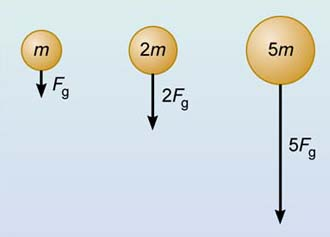Figure 1 Gravitational force is proportional to mass.

In fact, the gravitational force F g experienced by an object is proportional to its mass m (Figure 1), so we can write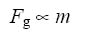Thus the force of gravity you experience due to the Earth is proportional to your own mass. Someone with a mass of 50 kg experiences only half the gravitational force felt by someone with a mass of 100 kg.

### Activity 3

How can we express this relationship between gravitational force and mass as an algebraic equation?

#### Discussion

We can change a proportionality relationship between two quantities into an equation by introducing a constant of proportionality.

In this case, the equation linking the gravitational force F g with the mass m that experiences the force can be written as: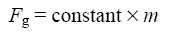To discover the meaning of the constant in this equation, let's consider the motion of an object of mass m that is falling towards the Earth, for example, an apple falling from a tree. Newton's second law of motion states that the acceleration a of the object is related to the unbalanced force F acting on it by the equation: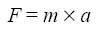We will assume that the force due to air resistance is very small, and can be neglected, so that the object falls freely, acted on by only the gravitational force F g. So the unbalanced force F in Equation 2 must be F g. Therefore we can rewrite Newton's second law for a freely-falling object as: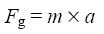We now have two equations for F g, 1 and 3, and so we can equate the right-hand sides as follows: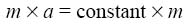If we divide both sides of this equation by m, this leads to an important conclusion about the acceleration a of an object acted on by the force of gravity alone: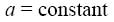The acceleration due to gravity experienced by any object that is falling freely close to the Earth's surface is a constant. It has the same value, irrespective of the mass of the object.

This was the surprising result discovered about 400 years ago by Galileo, who is alleged to have dropped two cannonballs of different mass from the top of the Leaning Tower of Pisa and observed that they took approximately the same time to reach the ground (Figure 2).Figure 2 Galileo's experiment, showing that cannonballs with different mass are accelerated equally by gravity. Historians doubt that Galileo performed this experiment, though he certainly did propose that gravity pulls large and small objects towards the Earth with the same acceleration. His proposal was so contentious at the time that he was forced to leave the University of Pisa.

The value of the acceleration due to gravity is always represented by the symbol g. It actually increases by about 0.5% between the Equator and the poles, and there are two reasons for this; one is the slightly non-spherical shape of the Earth and the other is the Earth's rotation. However, for the purpose of this course, we will usually ignore this small variation and will take the value of the acceleration due to gravity near to the Earth's surface to be 9.8 m s−2.

### Question 1

What is the force due to gravity acting on (a) a person of mass 80 kg, and (b) a 100 g bar of chocolate?

We know that when any object near the Earth's surface is acted on by the gravitational force alone, it has acceleration g=9.8 m s−2. So applying Newton's second law of motion, the gravitational force is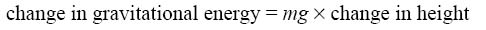1. For a person with m= 80 kg: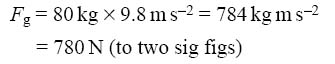2. For a 100 g bar of chocolate, we substitute m=0.10 kg into the equation F g=mg, and in this case the gravitational force is 0.98 N, which is about 1 N. {So if you want to know what 1 N feels like, pick up a 100 g object and experience the force that it exerts on your hand.}

The gravitational force F g experienced by an object is called the weight of the object. Now we showed that when an object is acted on by the force of gravity F g alone, then its acceleration is g, the acceleration due to gravity. So according to Newton's second law: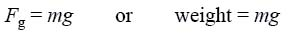Note that, whereas the mass m of an object is constant (provided it does not lose or gain matter), its weight F g is the gravitational force it experiences — and this depends on where on Earth (Figure 4.3) or in the Universe the object is located.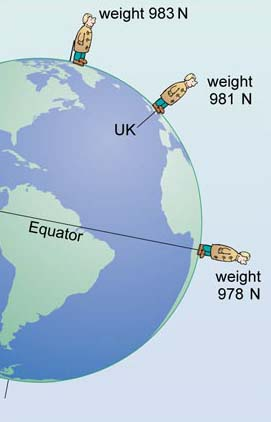Figure 3 The small variation of the acceleration due to gravity with latitude means that the weight of a 100 kg person depends on their location on Earth. At all three locations the person's weight is 980 N (and g=9.8 m s−2) to two significant figures.

### Question 2

What is the weight of a person with a mass of 80 kg (a) on the Earth (assuming g=9.8 m s−2), and (b) on the Moon where the acceleration due to gravity is roughly 1.6 ms−2?

(a) A person with mass m has weight mg. On Earth, g=9.8 m s−2, so the weight of an 80 kg person is 80 kg×9.8 ms−2=780 N (to two sig figs). {This is the same as the value you calculated in Question 1, since the weight of an object is the same as the gravitational force that acts on it. Next time you are asked your weight, you might like to give the value in newtons - and then explain to your friends the difference between the scientific and everyday use of the term weight!}

(b) On the Moon, g=1.6 m s−2, so in this case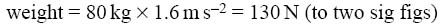{This shows that the weight of an object on the Moon is roughly one-sixth of its weight on Earth, even though its mass is the same in both places.}

## 2: Work done by gravity

It is possible to calculate the work done on a falling object by the gravitational force. We will adopt a simplifying approach and start by making the assumption that air resistance is negligible. In fact, air resistance is negligible for many practical purposes, so our calculations here won't be too unrealistic.

If an object falls a certain distance, work will be done on it by the gravitational force that is acting on it. This work will cause the kinetic energy of the object to increase as it falls. It is easy enough to calculate the energy involved. If we take as an example a book falling from a table onto the floor, as illustrated in Figure 4, we simply need to know the force (which is the weight of this book) and the distance travelled in the direction of the force (which is the height of the table).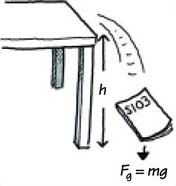Figure 4 A falling book.

### Activity 4

Suppose that this book has a mass m, and the table top is a distance h above the floor. Write down an equation for the work W done by gravity on the book as it falls from the table top to the floor.

#### Discussion

Work done W=Fd. In this case the force on the book is the weight, so F=F g=mg, and the distance moved is the table height, so d=h. Thus: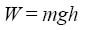### Question 3

1. Estimate the work done on this book by gravity if you let it fall from your table to the floor. (Box 1, Estimating, contains advice on how to tackle this sort of question.)

2. Assuming that air resistance is negligible, what is the kinetic energy of the book just before it hits the floor, and what is its speed at this point?

3. What happens to this kinetic energy when the book hits the floor?

1. The work done by gravity is W=mgh. You were asked to estimate the work done, so you need to estimate values for the mass of this book and for the height of your table. My estimates were m=0.1 kg and h=0.8 m. With these values, and using a value for g of 10 m s−2, the work done on the book by gravity as it falls is: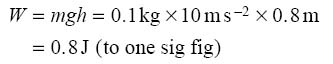{Your estimated values may have been somewhat different, but your answer shouldn't differ from mine by more than a factor of two. If it does, think again about your estimated values.}

2. The kinetic energy just before impact will be equal to the work done, so it is 0.8 J too. We can work out the speed from this kinetic energy by using the following equation,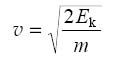and substituting Ek=0.8 J and m=0.1 kg. So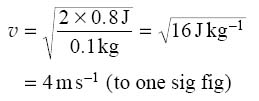{The SI unit of speed is m s−1, and we can show that this is equivalent to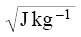as follows: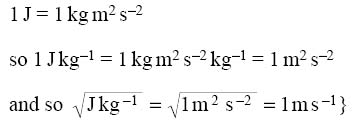3. The kinetic energy will be zero after the book comes to rest on the floor. However, energy is always conserved, so the kinetic energy must be converted into other forms of energy, in particular, to sound energy and internal energy of the book and the floor.

{Sound is transmitted as a pressure wave. As sound waves spread out, the molecules in the air collide with each other and with molecules of objects that they strike, and the energy transported by the sound wave is eventually converted into internal energy.}

### Box 1: Estimating

In Question 3(1), you are asked to 'estimate' the work done when a book falls to the floor and to do this you need to use estimates of the mass of the book and the height of the table. That means you are not expected to use exact measured values, but rather to make educated guesses. For example, you might pick up a book and think how its mass compares with that of a 1 kg bag of sugar, or a 100 g pot of yoghurt, or the mass of some other object. Similarly you could compare the height of the table with your own height. You would also round the value of g to 10 m s−2, since your estimates of mass and height will only justify quoting the estimated work done to one significant figure.

Estimating is a useful skill to acquire as it gives you practice at doing rough calculations. By making sensible estimates with approximate values you can get a feel for the size of units like the joule and the newton. It is often useful to check the computations of your calculator (and the accuracy of your key pressing) by making rough calculations with approximate values.

## 3: Work done against gravity - gravitational potential energy

So far we have only considered objects falling under gravity. Let's now consider the work done when we lift an object. In order to lift an object that has mass m, we have to apply an upward force mg to overcome the downward force of gravity. If this force raises the object through a height h, then the work done is: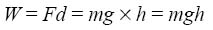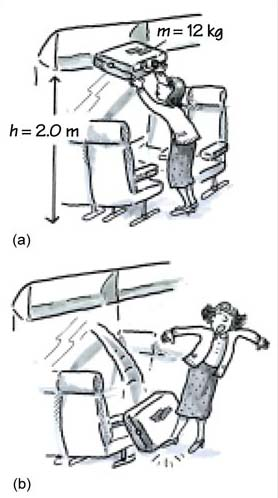Figure 5 (a) Placing a suitcase on a luggage rack involves doing work against gravity. (b) The stored energy is released if the suitcase falls off the rack.

So if an object of mass m is raised through a height h, the work done on the object is equal to mgh, and so this amount of energy is transferred to the object. (Notice that this equation is identical to the one describing an object falling under gravity, Equation 7.)

Of course, this ties in very well with everyday observations. If you lift a heavy suitcase onto a luggage rack in a train, or a heavy bag of shopping onto a table, you are very aware that you are doing work against gravity. You will also be aware that more work is required to lift a more massive object, or the same object to a greater height, and these 'observations' are consistent with the work done being equal to mgh.

### Activity 5

Calculate the work done in lifting a 12 kg suitcase from floor level up to a luggage rack 2.0 m above the floor (Figure 5a).

#### Discussion

The work done is W=mgh, so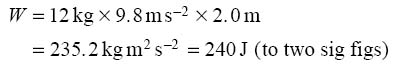You might wonder what has happened to the 240 J of energy that was supplied to the suitcase to lift it onto the luggage rack. According to the law of conservation of energy, energy can't just disappear. When work is done on a toy train, the energy supplied is converted into kinetic energy (and some internal energy when friction is taken into account), yet a suitcase placed on a luggage rack is obviously stationary. So where has the energy gone?

This question may be answered by considering what happens if the suitcase falls from the rack. As it falls, the energy reappears as kinetic energy, and the instant before it hits the floor all of the 240 J will be present as kinetic energy (Figure 5b). In other words, when the suitcase was lifted, energy was stored, ready to be released as kinetic energy when the case falls back down.

In general, when an object is raised to a greater height, work is done on the object and the energy transferred is stored; the amount of energy stored is mgfch, where Ah is the change in height. This stored energy is given the name of gravitational potential energy. The term 'potential' signifies that this energy has the 'potential' for doing work when the object is lowered. However, as gravitational potential energy is a bit longd-winded we will usually omit the 'potential' and refer to this energy just as gravitational energy E g. You will meet other forms of potential energy later in this block.

Now in all problems that involve gravitational energy it is the changes of gravitational energy that are important. A change of height leads to a change of gravitational energy; the absolute value of the gravitational energy is unimportant. So if we drop our 12 kg suitcase through 2 m in a room at the top of a tower block, it will have the same kinetic energy, and the same speed, just before it hits the floor as if it falls 2 m from the luggage rack to the floor of a train. And in neither case can we say that the suitcase has no gravitational energy after falling, since we could push it off a balcony, or out of the train, and its gravitational energy would decrease as it fell further.

We can emphasise the importance of these changes by writing an equation for gravitational energy in terms of changes. Thus:or using the Δ (delta) notation: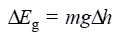Remember that ΔE g means 'the change in E g', and Δh means 'the change in h' Clearly when an object is raised to a greater height, its gravitational energy increases, and when it falls to a lower height, then its gravitational energy decreases.

## 4: Gravitational energy and energy conservation

The concept of gravitational energy greatly simplifies calculations concerned with the effect of gravity on the motion of objects, particularly where no other forces are concerned. For example, if you take your book (which by now is getting rather dogeared) and throw it vertically upwards in the air, it will slow down as it travels higher, and eventually reach a point where it is momentarily stationary. It will then accelerate downwards to where you (hopefully) catch it before it hits the ground. By using the idea of the change in gravitational energy, coupled with the law of conservation of energy, we can calculate such quantities as the speed of the book at a given height and the maximum height it will reach. These calculations assume that no air resistance acts on the book after it is thrown, so, in accordance with the law of conservation of energy, the sum of its kinetic energy and its gravitational energy must be constant. To say the same thing in another way — any increase in gravitational energy will be accompanied by a decrease in kinetic energy of equal size, and vice versa.

This will become clearer if we take a specific example. A book of mass m is thrown vertically upwards with an initial speed u. After it has risen through a height Δh it has a speed v.

### Activity 6

What is the increase in gravitational energy of the book?

#### Discussion

From Equation 8, the increase in gravitational energy is equal to mgΔh.

### Activity 7

What is the decrease in kinetic energy of the book?

#### Discussion

The book's initial kinetic energy is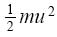and its final kinetic energy is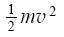; so the decrease in kinetic energy is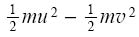.

(Note that this is a decrease in kinetic energy, since the initial speed u is greater than the final speed v.)

Since energy is conserved, the increase in gravitational energy must be equal to the decrease in kinetic energy, so we can write down the following equation: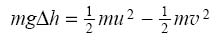Of course this equation doesn't apply only to books; it also applies to the movement of any object when gravity is the only force acting on it.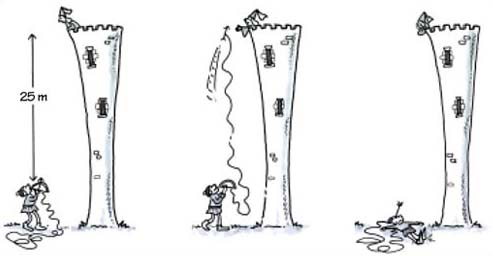Figure 6 An unsuccessful attempt to rescue a damsel in distress.

### Question 4

1. An archer is trying to rescue a damsel in distress by firing an arrow, with a rope attached, vertically upwards to her lonely turret at a height of 25 m above him (Figure 6). Calculate the speed with which the arrow must be released to just reach the damsel. (To simplify things, you should assume that the rope is a magic one, which is very strong but has no mass so that its energy changes can be ignored!)

2. If the damsel fails to catch the arrow, calculate the speed it will have when it returns to its starting point.

1. In this question we have to calculate the initial speed of the arrow, and all we are told is the height, 25 m, that it must reach. The arrow will just reach the damsel if the maximum height it reaches is 25 m, and at this point the arrow will have zero speed for an instant, and zero kinetic energy. So all of its initial kinetic energy has been converted into gravitational energy when it reaches this point, assuming there is no air resistance. The initial kinetic energy is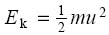, where u is the initial speed, and the increase in gravitational energy is ΔEg=mgΔh, where Δh is the maximum height. Since energy is conserved we can equate these two terms: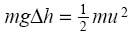We want to calculate the value of u, so we need to make u the subject of this equation. We first multiply both sides by 2 and divided both by m: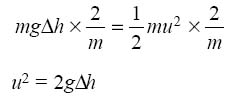and then take the square root of both sides: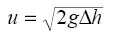If the values of g and Δh are substituted into the equation, we find: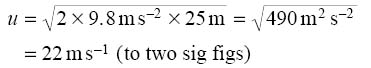2. As the arrow falls, the stored gravitational energy will be converted back into kinetic energy, and if it falls 25 m then its kinetic energy will be the same as that with which it started. The arrow's speed will therefore be the same as the speed with which it was released, that is 22 m s−1.

### Box 2: Manipulating algebra in problems

In the answer to Question 4, you may have noticed that we started with the equation: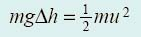and rearranged it to give: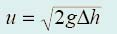before substituting the numerical values. This strategy of combining and rearranging equations to make the required quantity the subject before substituting numerical values was recommended in Question 4, and it is good practice in algebraic problems for the following reasons.

1. It is easier to see mistakes. If you substitute a lot of numbers and units into the original equation, and then try to rearrange it, it is very easy to lose track of where you are in the calculation. If you make an error, it is often very difficult to spot. When you do the rearrangement using the algebraic symbols, it is much easier to trace back through your answer and identify any errors.

2. It is easier to see how one quantity depends on another. For example, in Question 4, you can see that the initial speed is proportional to the square root of the maximum height.

3. Some symbols or numbers may cancel out when equations are rearranged, thus simplifying the final calculation. For example, the ms cancelled out in Question 4.

4. Having arrived at the final algebraic equation, it is very easy to repeat the calculation with different numerical values. If you substitute your numerical values first, you have to start the calculation afresh every time.

So you should generally rearrange algebraic equations first, and only substitute numerical values as the last stage of a calculation. Substituting numerical values earlier is not wrong, and if you do this you can still get the correct answer. However, it is best to substitute later if you can, for all of the reasons given above.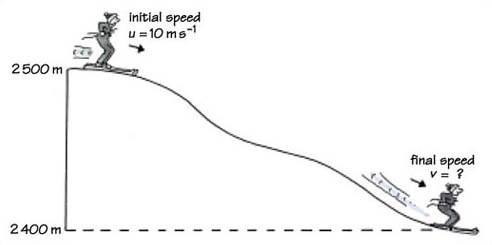Figure 7 The path followed by the skier in Question 5.

### Question 5

A skier starts on a downhill run from an altitude of 2 500 m with an initial speed of 10 m s−1 and follows the path shown in Figure 7 until she reaches an altitude of 2 400 m. What is her final speed at the end of the run, assuming air resistance and friction between the skis and the snow can be neglected?

We need to calculate the final speed of the skier, and we are given the initial and final altitudes, and the initial speed. If we assume friction and air resistance are negligible, the sum of the skier's kinetic energy and gravitational energy must remain constant throughout the run. So the gravitational energy that she loses as she descends is all converted into kinetic energy. The loss of gravitational energy is ΔEg=mgΔh, where Δh is the 100 m difference between the initial and final altitudes. The increase in kinetic energy is the difference between the kinetic energy at the finish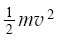and the kinetic energy at the start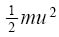. So: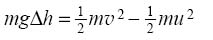{Note that the skier's speed is increasing, so v is greater than u, and the increase in kinetic energy must be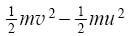, which has a positive value, rather than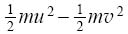We want to know the final speed v, so the equation needs some rearrangement. We first divide both sides by m, and this means that on the right we have to divide both |mv 2 and |mu 2 by m; this cancels all of equation: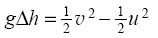Then multiply both sides by 2: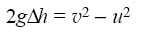and add u2 to both sides, so that v2 is left on its own on the right: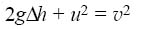We can now interchange the two sides of the equation and substitute the values given in the question: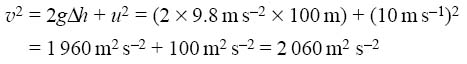{In substituting the values at this point in the calculation, you may feel we have not followed the good practice set out in Box 2. However, in cases such as this, it is often easier to work out v2 to save having to deal with several terms under a square root sign.}

Finally, we take the square root of both sides to get the value of v: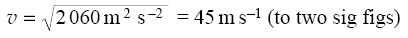{This is approximately 100 miles per hour! In reality, the final speed would be much less due to the combined effects of friction between the skis and snow, and air resistance. Notice that you didn't have to consider the complexities of the ski path to answer this question. Because of the law of conservation of energy, all you needed to know were the initial and final heights, or, to be more specific, the difference between the initial and final heights.}

Another common example of conversion between kinetic energy and gravitational energy is a child's swing, as illustrated in Figure 8a.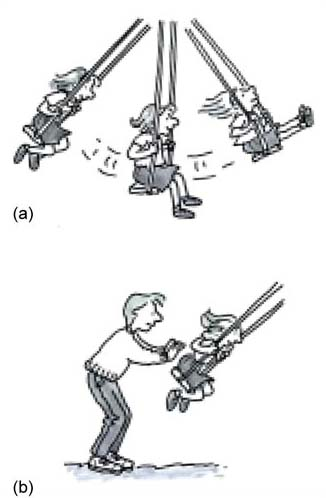Figure 8 (a) Motion of a swing during its swing cycle. The swing is shown at the two highest points of its motion and at the lowest point, (b) An extra energy input is needed to combat the effects of friction.

### Activity 8

At which point(s) during the motion of the swing will the gravitational energy have a maximum value, and at which point(s) will it have a minimum value?

#### Discussion

The gravitational energy must be at a maximum at the two highest points of the swing cycle and at a minimum at the lowest point of the cycle (these points are shown in Figure 8a).

### Activity 9

At which points(s) during the motion of the swing will the kinetic energy have a minimum value, and at which point(s) will it have a maximum value?

#### Discussion

Kinetic energy will be at a minimum value when the speed is at a minimum. This will occur at the highest points in the swing cycle, where the swing stops momentarily before reversing direction. Conversely, kinetic energy will be at a maximum when the speed is greatest - which is at the lowest point in the swing cycle shown in Figure 8a.

Alternatively we can use the law of conservation of energy to answer the previous question. If friction and air resistance are negligible, then the total energy is the sum of the kinetic energy and the gravitational energy, and this must be constant because energy is conserved. So the kinetic energy will be at a maximum when the gravitational energy is at a minimum and vice versa. This leads to exactly the same conclusion that we reached based on our experience of how the speed of a swing varies.

If there is no friction or air resistance gradually converting the available energy into internal energy, the swing would go backwards and forwards for ever, converting gravitational energy into kinetic energy and back again. However, for any real swing, there is always friction at the point of suspension, as well as air resistance during the swing's motion. Thus some of the kinetic energy will be converted into internal energy in the swing and its surroundings. For this reason a swing always comes to a halt in the absence of external forces - which is why parents are often employed to provide extra energy to maintain the swing's motion (Figure 8b).

The above discussion applies equally well to the motion of a pendulum. The conversion of kinetic energy into internal energy due to frictional forces can be made relatively very small by using a pendulum bob with a very large mass and suspending it from a very long cable.Figure 9 This huge pendulum bob was suspended from the dome of St. Paul's Cathedral in London for an Open University television programme. Presented by Professor Mike Pentz, who is seen on the left and was first Dean of Science at the University, the programme demonstrated that the plane in which the pendulum swung actually rotated relative to the Cathedral, because of the daily rotation of the Earth. Using a massive pendulum bob reduced the proportion of the energy of the pendulum that was converted into internal energy by air resistance forces during each swing, so the pendulum continued to swing freely for a long time.

### Question 6

Figure 9 shows an enormous pendulum in St. Paul's Cathedral, London. The pendulum bob had a mass of 80 kg, the suspension cable was 82 m long, and the bob swung back and forth through a distance of 6 m. If the height difference between the highest and lowest points in the bob's motion is 5.5 cm, calculate (a) the change in gravitational energy of the bob between these points, and (b) the maximum speed reached by the bob.

1. The change in gravitational energy is calculated from ΔEg=mgΔh (Equation 8), so substituting the values of mass (m=80 kg) and height difference (Δh=5.5 cm=0.055 m) quoted in the question, we find: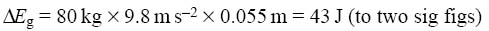2. The speed of the bob is zero at the highest point of the swing, so its kinetic energy is zero there too. As the bob swings down, gravitational energy is converted into kinetic energy, and the kinetic energy will be greatest at the lowest point, where the gravitational energy has its lowest value. This will therefore be the point where the speed has its maximum value.

Assuming that air resistance is negligible, conservation of energy tells us that the maximum kinetic energy of the pendulum is equal to the decrease in its gravitational energy. So: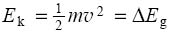If we multiply both sides by 2 and divide by m, we get: 2ΔE g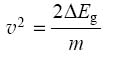{Once again, it is easier to substitute the values into the equation before finding the square root.} As Δ E g=43 J and m=80 kg: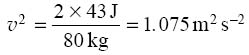If we take the square root of this value: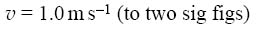{Note that the information about the length of the cable and the distance through which the bob swings was not needed to answer this question, but was included for interest, and to give you practice in selecting the information needed to answer questions.}

## 5: Other forms of potential energy

It is worth emphasising that the gravitational energy (or gravitational potential energy, to give it the full name) of an object increases when it moves in the opposite direction to the gravitational force (i.e. when it moves upwards). This relationship between energy and force does not apply only to gravity. For many forces, motion against the force (that is, in the opposite direction to the force) allows energy to be stored, and this energy can be reclaimed when the object is allowed to move in the direction of the force. We refer to these types of energy as potential energy because the object has the potential to do work if it is allowed to move in the direction of the force.

An important example of potential energy is the energy stored within an object when it is stretched, or squashed or otherwise deformed. This kind of potential energy is called strain potential energy (or strain energy for short), and it finds uses in all kinds of devices from the spring in a clock to the rubber band in a catapult.

If you compress a spring, you do work against the force that the spring exerts to try and maintain its natural length. The energy stored in the spring depends on the change in length of the spring - or, alternatively, on the distance that the end of the spring is moved relative to its natural, undeformed position (see Figure 10). When you release the spring, the stored strain energy is converted into kinetic energy.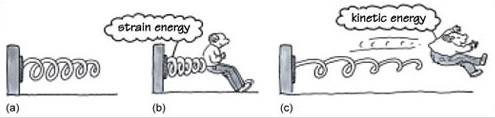Figure 10 (a) A spring in its natural, undeformed state. (b) When work is done to compress the spring, energy is stored in the spring as strain energy. (c) When the spring is released, strain energy is converted into kinetic energy.

The distinguishing feature of potential energy in all its forms is that it depends on the position of an object and not on its motion. Thus gravitational (potential) energy depends on the height of an object, and strain (potential) energy depends on the compression (or extension) of an object.

## Conclusion

All objects, irrespective of their mass, experience the same acceleration g when falling freely under the influence of gravity at the same point on the Earth. Close to the Earth's surface, g=9.8 m s−2. The weight of an object is the force F g due to gravity acting on the object, and for an object with mass m the weight is given by F g=mg.

If the height of an object of mass m changes by Δh, the change in gravitational energy is ΔE g=mgΔh.

If gravity is the only force acting on an object, the sum of kinetic energy and gravitational energy is constant. Increases in kinetic energy are balanced by decreases in gravitational energy, and vice versa.

There are various forms of potential energy, all of which depend on the position of an object rather than on its motion. The potential energy of an object increases as it moves in the opposite direction to that of the force acting on it. Strain energy depends on the extension or compression of an object.

## Acknowledgements

Course image: Bureau of Land Management Oregon and Washington in Flickr made available under Creative Commons Attribution-NonCommercial-ShareAlike 2.0 Licence.

The content acknowledged below is Proprietary (see terms and conditions) and is used under licence.

All other materials included in this course are derived from content originated at the Open University.

Don't miss out:

If reading this text has inspired you to learn more, you may be interested in joining the millions of people who discover our free learning resources and qualifications by visiting The Open University - www.open.edu/ openlearn/ free-courses